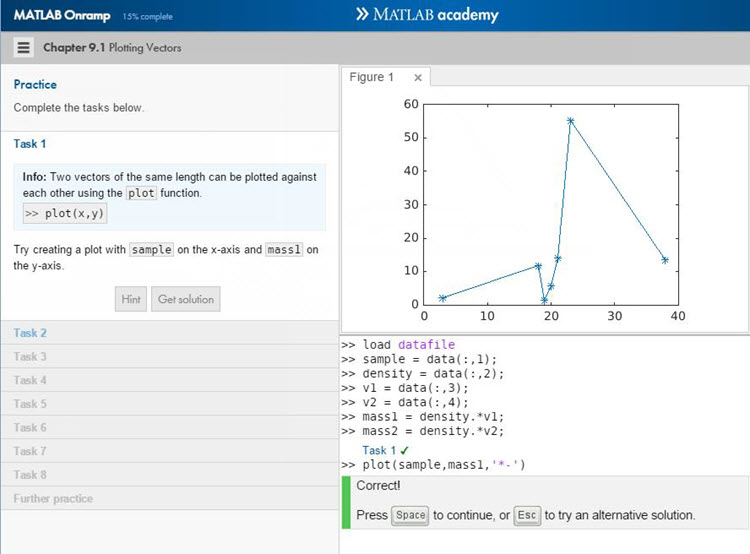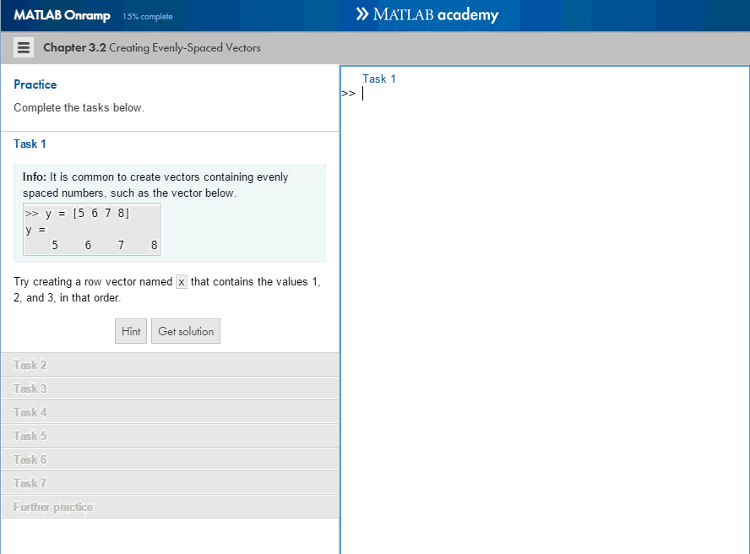# MATLAB Onramp

In the last post, Wendy Fullam told us about MATLAB Examples, where you could see working examples and pick apart the code to see how they worked. But suppose you wanted more? Suppose you wanted an environment that could teach you, step by step, how to get started coding in MATLAB? If that’s the case, then Chaitanya Chitale has just what you need. Chaitanya is a MATLAB Training Content Developer at MathWorks.

# Introducing MATLAB Onramp

by Chaitanya Chitale

Are you new to MATLAB and looking for a way to get started? If so, check out the new MATLAB Onramp course.

MATLAB Onramp is an online course that provides a brief introduction to the MATLAB language. The course gives you hands-on MATLAB experience via the use of an integrated, web-based version of MATLAB, as shown below.As you work through the course you can try out different solutions to the problems asked and get immediate feedback.## Course content and duration

The MATLAB Onramp course covers the basics of importing data, manipulating arrays, creating visualizations, and much more.

The course takes approximately 2 hours to complete. However, you can take the course at your own pace. After starting it, you can leave and come back to it at any time.